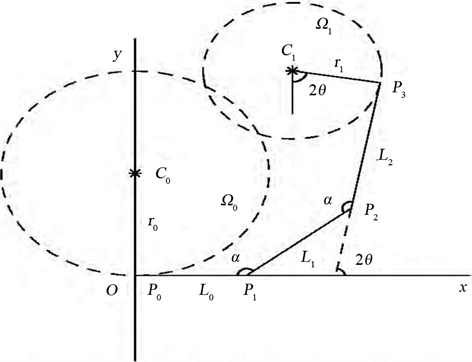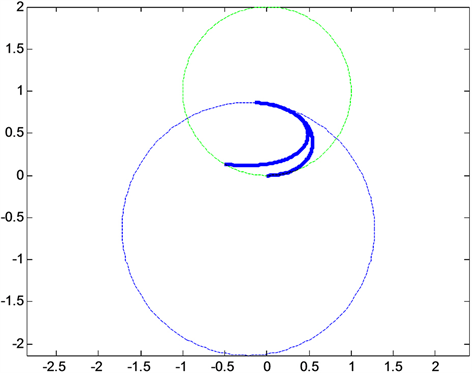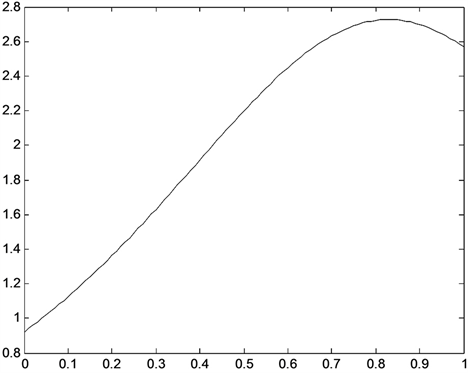﻿ 三次PH-DP过渡曲线的构造

# 三次PH-DP过渡曲线的构造Construction of Cubic PH-DP Transition Curve

Abstract: Based on the geometric characteristics of the DP curve as the PH curve, this chapter uses the cubic PH-DP curve to construct a C-shaped transition curve between two circles that do not contain each other, and gives numerical examples.

1. 引言

PH曲线的主要特征是具有有理形式的等距线和多项式弧长，这些优点使得PH曲线广泛应用于等距线生成、机器人行走路径设计、道路设计、数控机械加工中，随后又推广到有理形式的PH曲线。郑志浩，汪国昭  提出用三次PH曲线构造两曲率圆内含情况下的过渡曲线，并给出这种情况下圆心距的取值范围，取得了较好的结果。刘莹莹  提出用三次PH曲线构造两曲率圆相离情况下的过渡曲线，并给出这种情况下圆心距的取值范围。而在2003年，Delagdo和Pena提出一种新的参数曲线，称其基函数为 DP基，因此构造的曲线被称为DP曲线 。该曲线是由割角算法生成的，并且其基函数具有优于其他基函数的性质，因为它是保型性全正基(NTP基)，并在数值计算过程中具有稳定性，且其计算简便，计算复杂度为线性的。因此，DP曲线在计算速度方面优于Bézier曲线，并具有研究的意义。2019年，李尚蔚  基于DP曲线，构造了三次PH-DP曲线，并给出三次DP曲线成为PH曲线的控制多边形几何特征条件。本文通过PH-DP控制多边形几何特征条件，做过渡曲线，并给出数值例子。

2. DP曲线成为PH曲线的几何特征条件

2.1. 三次PH曲线

${B}_{i}^{n}\left(t\right)=\left(\begin{array}{l}n\\ i\end{array}\right){\left(1-t\right)}^{n-i}{t}^{i},0\le t\le 1$ 是Bernstein基函数，n次Bézier曲线定义为

$P\left(t\right)=\left(x\left(t\right),y\left(t\right)\right)=\underset{i=0}{\overset{n}{\sum }}{B}_{i}^{n}\left(t\right){P}_{i},$ (1)

${{x}^{\prime }}^{2}\left(t\right)+{{y}^{\prime }}^{2}\left(t\right)={\delta }^{2}\left(t\right),$

$P\left(t\right)$ 为n次Pythagorean-hodograph曲线，当 $n=3$ 时简称为3次PH曲线。PH曲线的特点是其弧长及等距线表达式为多项式或有理多项式。

2.2. DP曲线

$Q\left(t\right)=\underset{i=0}{\overset{3}{\sum }}{q}_{i}{D}_{i,3}\left(t\right)$ (2)

$\left[{D}_{0,3},{D}_{1,3},{D}_{2,3},{D}_{3,3}\right]=\left[{\left(1-t\right)}^{3},t\left(1-t\right)\left(2-t\right),t\left(1-t\right)\left(1+t\right),{t}^{3}\right]$

2.3. 三次PH-DP曲线的控制多边形特征

$\left\{\begin{array}{l}4L=9{L}_{1}{L}_{2}\\ {\theta }_{1}={\theta }_{2}\end{array}$ (3)Figure 1. Coordinate system setting and endpoint curvature diagram of cubic PH curve

$\begin{array}{l}{P}_{0}=\left({x}_{0},{y}_{0}\right)=\left(0,0\right),\\ {P}_{1}=\left({x}_{1},{y}_{1}\right)=\left({L}_{0},0\right),\\ {P}_{2}=\left({x}_{2},{y}_{2}\right)=\left({L}_{0}+{L}_{1}\mathrm{cos}\theta ,{L}_{1}\mathrm{sin}\theta \right),\\ {P}_{3}={P}_{4}=\left({x}_{3},{y}_{3}\right)=\left({L}_{0}+{L}_{1}\mathrm{cos}\theta +{L}_{2}\mathrm{cos}2\theta ,{L}_{1}\mathrm{sin}\theta +{L}_{2}\mathrm{sin}2\theta \right).\end{array}$ (4)

3. 三次PH-DP过渡曲线的构造

$P\left(t\right)=\left(x\left(t\right),y\left(t\right)\right)={P}_{0}{\left(1-t\right)}^{3}+{P}_{1}t\left(1-t\right)\left(2-t\right)+{P}_{2}t\left(1-t\right)\left(1+t\right)+{P}_{3}{t}^{3}$

$\begin{array}{l}x\left(t\right)={L}_{0}t\left(1-t\right)\left(2-t\right)+\left({L}_{0}+{L}_{1}\mathrm{cos}\theta \right)t\left(1-t\right)\left(1+t\right)+\left({L}_{0}+{L}_{1}\mathrm{cos}\theta +{L}_{2}\mathrm{cos}2\theta \right){t}^{3}\\ y\left(t\right)={L}_{1}\mathrm{sin}\theta t\left(1-t\right)\left(1+t\right)+\left({L}_{1}\mathrm{sin}\theta +{L}_{2}\mathrm{sin}2\theta \right){t}^{3}\end{array}$

$k\left(t\right)=\frac{\sqrt{{L}_{0}{L}_{2}}\mathrm{sin}\theta }{{\left(2{L}_{0}{\left(1-t\right)}^{2}+3\sqrt{{L}_{0}{L}_{2}}\mathrm{cos}\theta t\left(1-t\right)+2{L}_{2}{t}^{2}\right)}^{2}}$

$t=0,1$ 带入

$\begin{array}{l}{k}_{0}=k\left(0\right)=\frac{\sqrt{{L}_{0}{L}_{2}}\mathrm{sin}\theta }{4{L}_{0}^{2}}\\ {k}_{1}=k\left(1\right)=\frac{\sqrt{{L}_{0}{L}_{2}}\mathrm{sin}\theta }{4{L}_{2}^{2}}\end{array}$

$0<\theta <\frac{\pi }{2}$ 时， ${k}_{0}$${k}_{1}$ 同号，即两圆互不包含时的过渡曲线是C的，对曲率求导，得到PH-DP曲线的曲率导数为

${k}^{\prime }\left(t\right)=\frac{6\sqrt{{L}_{0}{L}_{2}}\mathrm{sin}\theta \left(\left(\sqrt{{L}_{0}{L}_{2}}\mathrm{cos}\theta -\frac{4}{3}{L}_{0}\right)\left(1-t\right)+\left({L}_{2}-\sqrt{{L}_{0}{L}_{2}}\mathrm{cos}\theta \right)t\right)}{2{L}_{0}{\left(1-t\right)}^{2}+3\sqrt{{L}_{0}{L}_{2}}\mathrm{cos}\theta t\left(1-t\right)+2{L}_{2}{t}^{2}}$

$t=0,1$ 带入

$\begin{array}{l}{k}^{\prime }\left(0\right)=\frac{-6\sqrt{{L}_{0}{L}_{2}}\mathrm{sin}\theta \left(\sqrt{{L}_{0}{L}_{2}}\mathrm{cos}\theta -\frac{4}{3}{L}_{0}\right)}{8{L}_{0}^{3}}\\ {k}^{\prime }\left(1\right)=\frac{-6\sqrt{{L}_{0}{L}_{2}}\mathrm{sin}\theta \left({L}_{2}-\sqrt{{L}_{0}{L}_{2}}\mathrm{cos}\theta \right)}{8{L}_{2}^{3}}\end{array}$

$0<\lambda <1,0<\theta <\mathrm{arccos}\lambda$

${k}^{\prime }\left(0\right)\cdot {k}^{\prime }\left(1\right)<0$

$0.5176<\lambda <1$${r}_{0}-{r}_{1}

$q\left(\lambda \right)=\frac{\sqrt{9-8{\lambda }^{2}+14{\lambda }^{4}-8{\lambda }^{6}+9{\lambda }^{8}}}{3\left(1-{\lambda }^{4}\right)}$

${L}_{0}=\frac{1}{4}\lambda {r}_{0}\mathrm{sin}\theta$

${L}_{2}=\frac{1}{4}{\lambda }^{3}{r}_{0}\mathrm{sin}\theta$

$\begin{array}{c}g\left(m\right)=-\frac{1}{4}{\lambda }^{4}{r}_{0}^{2}{m}^{4}+\frac{13}{16}{\lambda }^{3}{r}_{0}^{2}\left(1+{\lambda }^{2}\right){m}^{3}+\frac{{\lambda }^{2}}{16}{r}_{0}^{2}\left(-1+11\lambda -\frac{25}{4}\right){m}^{2}-\frac{13}{16}{\lambda }^{3}\left(1+{\lambda }^{2}\right)m\\ \text{\hspace{0.17em}}\text{\hspace{0.17em}}+\frac{1}{64}\left(-64{r}^{2}+\left(64-44{\lambda }^{2}+9{\lambda }^{4}-44{\lambda }^{6}+64{\lambda }^{8}\right){r}_{0}^{2}\right)\\ =0\end{array}$

$g\left(0\right)=\frac{1}{64}\left(-64{r}^{2}+\left(64-44{\lambda }^{2}+9{\lambda }^{4}-44{\lambda }^{6}+64{\lambda }^{8}\right){r}_{0}^{2}\right)$

$g\left(\lambda \right)=\left(1-\frac{1}{16}{\lambda }^{2}-\frac{43}{64}{\lambda }^{4}+\frac{299}{64}{\lambda }^{6}-\frac{9}{4}{\lambda }^{8}\right){r}_{0}^{2}-{r}^{2}$

$g\left(0\right)=\frac{64-44{\lambda }^{2}+9{\lambda }^{4}-44{\lambda }^{6}+64{\lambda }^{8}}{64{\left(1-{\lambda }^{4}\right)}^{2}}{\left({r}_{0}-{r}_{1}\right)}^{2}-{r}^{2}$

$g\left(\lambda \right)=\frac{43+299{\lambda }^{2}-144{\lambda }^{4}}{64{\left(1+{\lambda }^{2}\right)}^{2}}{\left({r}_{0}-{r}_{1}\right)}^{2}-{r}^{2}$

$0<\lambda <1$$0<\frac{\sqrt{43+299{\lambda }^{2}-144{\lambda }^{4}}}{8\left(1+{\lambda }^{2}\right)}<1$。因为 $r>{r}_{0}-{r}_{1}$，所以 $g\left(\lambda \right)<0$。由此得 $g\left(0\right)>0$

$g\left(0\right)>0$

$\frac{\sqrt{64-44{\lambda }^{2}+9{\lambda }^{4}-44{\lambda }^{6}+64{\lambda }^{8}}}{8\left(1-{\lambda }^{4}\right)}\left({r}_{0}-{r}_{1}\right)<\frac{\sqrt{9-8{\lambda }^{2}+14{\lambda }^{4}-8{\lambda }^{6}+9{\lambda }^{8}}}{3\left(1-{\lambda }^{4}\right)}\left({r}_{0}-{r}_{1}\right)Figure 2. The transition curve of the three times PH-DP at $\left({r}_{0}=1.5,{r}_{1}=1\right)$Figure 3. The curvature of the cubic PH-DP curve at $\left({r}_{0}=1.5,{r}_{1}=1\right)$

${g}^{\prime }\left(m\right)=-\frac{13}{16}{\lambda }^{3}\left(1+{\lambda }^{2}\right)+\frac{1}{8}{\lambda }^{4}\left(-\frac{25}{4}+11{\lambda }^{2}\right){h}^{2}+\frac{39}{16}{\lambda }^{3}\left(1+{\lambda }^{2}\right){h}^{2}-{\lambda }^{4}{h}^{3}$

${g}^{\prime }\left(0\right)=-\frac{13}{16}{\lambda }^{3}\left(1+{\lambda }^{2}\right)<0$

${g}^{″}\left(0\right)=\frac{1}{8}{\lambda }^{4}\left(-\frac{25}{4}+11{\lambda }^{2}\right){r}_{0}^{2}>0$${g}^{″}\left(m\right)=\frac{1}{32}{\lambda }^{4}\left(-99+104{\lambda }^{2}\right)>0$，所以 ${g}^{\prime }\left(m\right)$ 是一个单调递增函数。又因为在 $\left(0,\lambda \right)$$g\left(m\right)=0,{g}^{\prime }\left(m\right)<0$，所以 ${g}^{\prime }\left(m\right)$ 是一个单调递增函数。所以 $g\left(m\right)$ 有唯一根。

4. 数值例子

${r}_{0}=1.5$，由 $0.5176<\lambda <1$，取 ${r}_{1}=1$，这时起始圆的圆心为(0,1.5)，通过计算可得过渡曲线两端点的曲率圆的圆心距的范围 ${r}_{0}-{r}_{1}，取 $r=2.3$，解得 $\theta =1.0842$，则所生成的三次PH-DP过渡曲线如图2所示，过渡曲线的曲率如图3所示，其内部曲率极值点个数为1。

 Zboinski, K. and Woznica, P. (2017) Optimization of Polynomial Transition Curves from the Viewpoint of Jerk Value. Archives of Civil Engineering, 63, 181-199.
https://doi.org/10.1515/ace-2017-0012

 Li, Z., Ma, L.Z. and Meek, D. (2006) Reconstruction of G2 Transition Curve for Two Separated Circular Arcs. Journal of Computer-Aided Design & Computer Graphics, 18, 265-269.

 高晖, 寿华好. α-曲线的构造及曲率单调条件的研究[J]. 高校应用数学学报, 2015, 30(3): 291-305.

 高晖, 寿华好, 缪永伟, 等. 3个控制顶点的类三次Bézier螺线[J]. 中国图象图形学报, 2014, 19(11): 1677-1683.

 Meekd, S. and Waltond, J. (1989) The Use of Comuspirals in Drawing Planar Curves of Controlled Curvature. Journal of Computational and Applied Mathematics, 25, 69-78.
https://doi.org/10.1016/0377-0427(89)90076-9

 Waltond, J. and Meekd, S. (1996) A Planar Cubic Bézier Spiral. Journal of Computational and Applied Mathematics, 72, 85-100.
https://doi.org/10.1016/0377-0427(95)00246-4

 Walton, D.J. and Meekd, S. (1998) G2 Curves Composed of Planar Cubic and Pythagorean Hodographs Quintic Spirals. Computer Aided Geometric Design, 15, 547-566.
https://doi.org/10.1016/S0167-8396(97)00028-9

 Habib, Z. and Sakai, M. (2007) G2 Pythagorean Hodographs Quintic Transition between Circles with Shape Control. Computer Aided Geometric Design, 24, 252-266.
https://doi.org/10.1016/j.cagd.2007.03.004

 Habib, Z. and Sakai, M. (2007) On PH Quintic Spirals Joining Two Circles with One Circle inside the Other. Computer Aided Design, 39, 125-132.